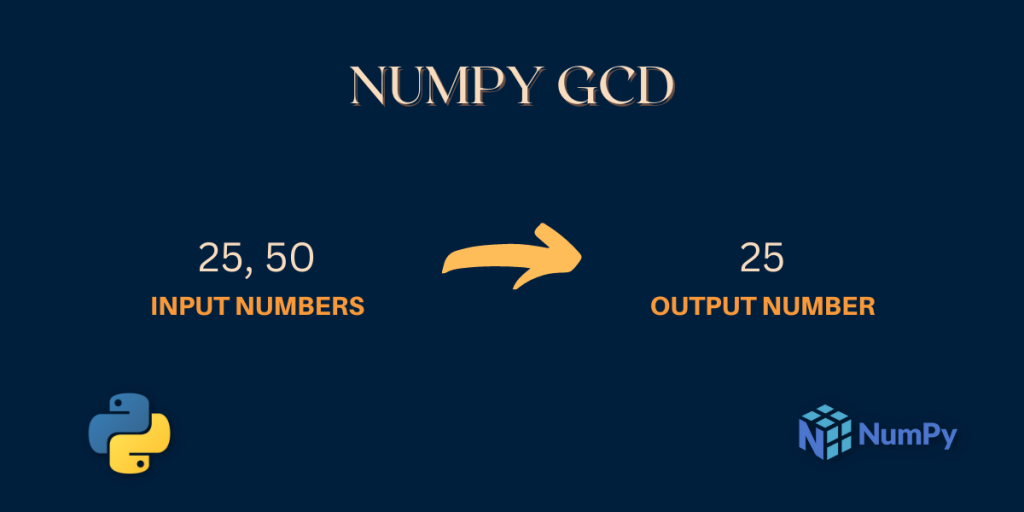# NumPy gcd – Returns the greatest common divisor of two numbersAs we saw in the previous exercise, NumPy’s lcm function is very useful for finding the least common multiple of two or more numbers What if we want the greatest common divisor of two or more numbers instead? That’s where the numpy gcd function comes into play. The Greatest Common Divisor (GCD) of two or more numbers is the largest common factor that divides the numbers exactly.

Also read: NumPy lcm – Returns the Lowest Common Multiple of two numbers

## What is NumPy gcd?

The greatest common divisor (GCD) of two integers is the largest integer that evenly divides both integers. For example, the GCD of 16 and 24 is 8, since 8 is the largest integer that evenly divides both 16 and 24.

NumPy gcd is a mathematical function that calculates the GCD value of the input numbers given to the `nunpy.gcd()` function.

## Syntax of NumPy gcd

Let’s look at the syntax of the function.

```numpy.gcd(x1 , x2)
```

The inputs `x1` , `x2` cannot be negative numbers.

Here, `x1` and `x2` are the input numbers that can be single numbers or a NumPy array of numbers. The `numpy.gcd()` function calculates the GCD (Greatest Common Divisor) of the input numbers.

Note: The numbers cannot be floating point numbers.

Let’s write some code to understand the function better.

## Working with NumPy gcd

The greatest common divisor of two integers can be calculated using NumPy’s gcd function. Here’s how it works:

### NumPy gcd of Single Numbers

```import numpy as np

print("The GCD of 12 and 46 is:",np.gcd(12 , 46))

print("The GCD of 12 and 24 is:",np.gcd(12 , 24))

print("The GCD of 25 and 50 is:",np.gcd(25 , 50))

print("The GCD of 5 and 100 is:",np.gcd(5 , 100))

print("The GCD of 17 and 87 is:",np.gcd(17 , 87))
```

### Output

```The GCD of 12 and 46 is: 2
The GCD of 12 and 24 is: 12
The GCD of 25 and 50 is: 25
The GCD of 5 and 100 is: 5
The GCD of 17 and 87 is: 1
```

In the above code snippet, we have imported the NumPy library using the `import` statement.

In the next lines, we are using the `np.gcd()` function to calculate the GCD of the numbers. Notice how fast we are getting the GCD of two numbers, that’s the power of the Python NumPy library 🙂

Let’s understand how the GCD of 25 and 50 is calculated. We can observe that the greatest number that divides both 25 and 50 is 25. So, the GCD of 25 and 50 is 25 which is also the output produced by the `np.gcd()` function.

Now, let us see how can we calculate the GCD of an array of numbers.

### NumPy gcd of a single NumPy array of Numbers

Here, we will find the gcd of all the elements of a single NumPy array.

```import numpy as np

a = np.array((3, 6, 24, 56, 79, 144))

gcd_value = np.gcd.reduce(a)

print("Input Array:\n",a)
print("The GCD value of the elements of the array is:",gcd_value)
```

### Output

```Input Array:
[  3   6  24  56  79 144]
The GCD value of the elements of the array is: 1
```

To find the GCD of all the elements of a single NumPy array the `reduce` function is used that applies the `gcd` method to each element of the array.

The rest of the work of the function is the same.

Can we calculate the GCD of two NumPy arrays? Let’s see 🙂

### NumPy gcd of two NumPy arrays of numbers

```import numpy as np

a = np.array((12 , 24 , 99))

b = np.array((44 , 66 , 27))

c = np.gcd(a , b)

print("Array 1:\n",a)
print("Array 2:\n",b)

print("GCD values:\n",c)
```

### Output

```Array 1:
[12 24 99]
Array 2:
[44 66 27]
GCD values:
[4 6 9]
```

That’s amazing! We just calculated the GCD of two NumPy arrays.

Here, the `np.gcd()` function picks the elements at the same positions in the two arrays and calculates their GCD. For example, in the above code snippet, 12 and 44 are selected and their GCD is calculated. Similarly, the next element from both arrays and their GCD is calculated.

The output of `np.gcd()` is a NumPy array that is stored in the variable `c` in the above code snippet.

So, that was all about that NumPy gcd function.

## Summary

In this article, we learned about the syntax of the function and practiced different types of examples that made our understanding clear. These NumPy functions are really simple to use and you know what this is the power of the NumPy library. It has got a lot of mathematical functions that make things easy when we are performing some mathematical calculations on large Data.

Keep exploring amazing articles on other Python Topics here.

## Reference

NumPy Documentation – NumPy gcd